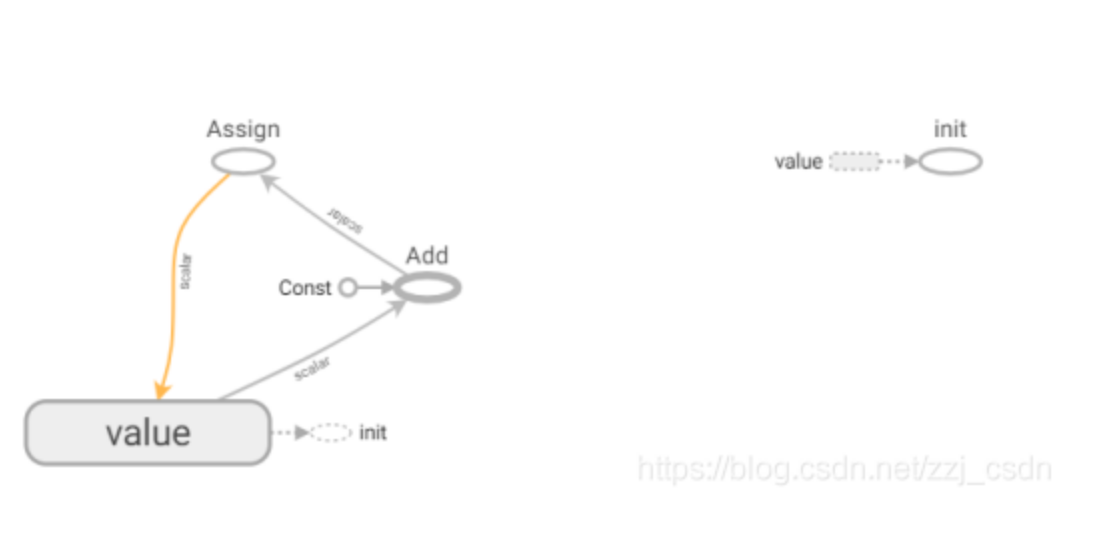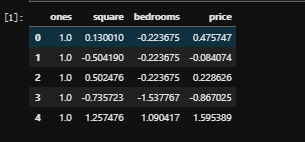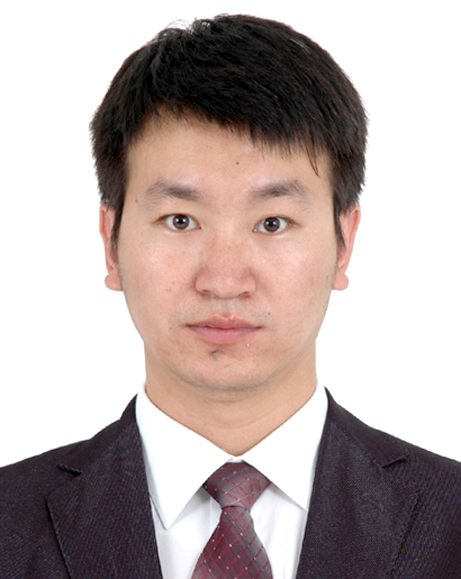# tensorflow实现基于深度学习的图像补全

+关注继续查看■ 第一步：将图像理解为一个概率分布的样本

你是怎样补全缺失信息的呢？

但是怎样着手统计呢？这些都是图像啊。

那么我们怎样补全图像？

■ 第二步：快速生成假图像

在未知概率分布情况下，学习生成新样本

[ML-Heavy] 生成对抗网络(Generative Adversarial Net, GAN) 的架构

使用G(z)生成伪图像

[ML-Heavy] 训练DCGAN

现有的GAN和DCGAN实现

[ML-Heavy] 在Tensorflow上构建DCGANs

在图片集上跑DCGAN

使用 DCGAN 进行图像补全

[ML-Heavy] 到 pgpg 的投影的损失函数

[ML-Heavy] 使用tensorflow来进行DCGAN图像补全

补全图像

■ 结论

• 首先我们将图像理解为一个概率分布的样本。

• 基于这种理解，学习如何生成伪图片。

• 然后我们找到最适合填充回去的伪图片。

1.你是怎样补全缺失信息的呢？

2.但是怎样着手统计呢？这些都是图像啊。2维图像的PDF和采样。 PDF 用等高线图表示，样本点画在上面。

RGB颜色 表示。我们的图像宽64像素，高64像素，所以我们的概率分布是 64⋅64⋅3≈12k 维的。

3.那么我们怎样补全图像？统计模型，然后拟合数据即可。

1.在未知概率分布情况下，学习生成新样本Yann LeCun 对生成对抗网络的介绍2.[ML-Heavy] 生成对抗网络(GenerativeAdversarial Net, GAN) 的架构

z =np.random.uniform(-1, 1, 5)

array([0.77356483,  0.95258473,-0.18345086,  0.69224724, -0.34718733])

def G(z):

...

return imageSample

z = np.random.uniform(-1,1, 5)

imageSample =G(z)DCGAN 论文也提出了其他的在训练 DCGANs 时的技巧和调整，比如批量正则化（batchnormalization）以及 leaky RELUs。

3.使用G(z)生成伪图像4.[ML-Heavy] 训练DCGAN·      1、对于真实数据分布x∼pdatax∼pdata的每一张图片，最大化D(x)。

·      2、对于不是真实数据分布x≁pdatax≁pdata的每一张图片，最小化D(x)。

minGmaxDEx∼pdatalog(D(x)+Ez∼pz[log(1−D(G(z)))]minGmaxDEx∼pdatalog(D(x)+Ez∼pz[log(1−D(G(z)))]

GAN 论文中的训练算法。

5.现有的GAN和DCGAN实现

goodfeli/adversarial: GAN论文作者写的 Theano GAN 实现。
tqchen/mxnet-gan: 非官方 MXNet GAN 实现。
Newmu/dcgan_code: DCGAN论文作者写的 Theano GAN 实现。
soumith/dcgan.torch: DCGAN论文作者之一 (Soumith Chintala) 的 Torch DCGAN 实现。
carpedm20/DCGAN-tensorflow: 非官方 TensorFlow DCGAN 实现。
openai/improved-ganOpenAI 第一篇论文背后的代码。在 carpedm20/DCGAN-tensorflow 基础上进行了大量的修改。
mattya/chainer-DCGAN: 非官方 Chainer DCGAN 实现。
jacobgil/keras-dcgan: 非官方 (未完成) KerasDCGAN 实现。

6.[ML-Heavy] 在Tensorflow上构建DCGANs

defgenerator(self, z):

self.z_, self.h0_w, self.h0_b = linear(z,self.gf_dim*8*4*4, 'g_h0_lin', with_w=True)

self.h0 = tf.reshape(self.z_, [-1, 4, 4,self.gf_dim * 8])

h0 = tf.nn.relu(self.g_bn0(self.h0))

self.h1, self.h1_w, self.h1_b =conv2d_transpose(h0,

[self.batch_size, 8, 8, self.gf_dim*4],name='g_h1', with_w=True)

h1 =tf.nn.relu(self.g_bn1(self.h1))

h2, self.h2_w, self.h2_b =conv2d_transpose(h1,

[self.batch_size, 16, 16,self.gf_dim*2], name='g_h2', with_w=True)

h2 = tf.nn.relu(self.g_bn2(h2))

h3, self.h3_w, self.h3_b =conv2d_transpose(h2,

[self.batch_size, 32, 32,self.gf_dim*1], name='g_h3', with_w=True)

h3 = tf.nn.relu(self.g_bn3(h3))

h4, self.h4_w, self.h4_b =conv2d_transpose(h3,

[self.batch_size, 64, 64, 3],name='g_h4', with_w=True)

return tf.nn.tanh(h4)

defdiscriminator(self, image, reuse=False):

if reuse:

tf.get_variable_scope().reuse_variables()

h0 = lrelu(conv2d(image, self.df_dim,name='d_h0_conv'))

h1 = lrelu(self.d_bn1(conv2d(h0,self.df_dim*2, name='d_h1_conv')))

h2 = lrelu(self.d_bn2(conv2d(h1,self.df_dim*4, name='d_h2_conv')))

h3 = lrelu(self.d_bn3(conv2d(h2,self.df_dim*8, name='d_h3_conv')))

h4 = linear(tf.reshape(h3, [-1, 8192]), 1,'d_h3_lin')

return tf.nn.sigmoid(h4), h4

self.G =self.generator(self.z)

self.D,self.D_logits = self.discriminator(self.images)

self.D_,self.D_logits_ = self.discriminator(self.G, reuse=True)

self.d_loss_real= tf.reduce_mean(

tf.nn.sigmoid_cross_entropy_with_logits(self.D_logits,

tf.ones_like(self.D)))

self.d_loss_fake= tf.reduce_mean(

tf.nn.sigmoid_cross_entropy_with_logits(self.D_logits_,

tf.zeros_like(self.D_)))

self.g_loss =tf.reduce_mean(

tf.nn.sigmoid_cross_entropy_with_logits(self.D_logits_,

tf.ones_like(self.D_)))

self.d_loss =self.d_loss_real + self.d_loss_fake

t_vars = tf.trainable_variables()

self.d_vars =[var for var in t_vars if 'd_' in var.name]

self.g_vars =[var for var in t_vars if 'g_' in var.name]

d_optim =tf.train.AdamOptimizer(config.learning_rate, beta1=config.beta1) \

.minimize(self.d_loss,var_list=self.d_vars)

g_optim =tf.train.AdamOptimizer(config.learning_rate, beta1=config.beta1) \

.minimize(self.g_loss,var_list=self.g_vars)

for epoch inxrange(config.epoch):

...

for idx in xrange(0, batch_idxs):

batch_images = ...

batch_z = np.random.uniform(-1, 1,[config.batch_size, self.z_dim]) \

.astype(np.float32)

# Update D network

_, summary_str =self.sess.run([d_optim, self.d_sum],

feed_dict={ self.images:batch_images, self.z: batch_z })

# Update G network

_, summary_str =self.sess.run([g_optim, self.g_sum],

feed_dict={ self.z: batch_z })

# Run g_optim twice to make sure thatd_loss does not go to zero (different from paper)

_, summary_str =self.sess.run([g_optim, self.g_sum],

feed_dict={ self.z: batch_z })

errD_fake =self.d_loss_fake.eval({self.z: batch_z})

errD_real =self.d_loss_real.eval({self.images: batch_images})

errG = self.g_loss.eval({self.z:batch_z})

7.在图片集上跑DCGAN

git clonehttps://github.com/cmusatyalab/openface.git

git clonehttps://github.com/bamos/dcgan-completion.tensorflow.git

./openface/util/align-dlib.pydata/dcgan-completion.tensorflow/data/your-dataset/raw aligninnerEyesAndBottomLipdata/dcgan-completion.tensorflow/data/your-dataset/aligned --size 64

cddcgan-completion.tensorflow/data/your-dataset/aligned

find . -name'*.png' -exec mv {} . \;

find . -type d-empty -delete

cd ../../..

./train-dcgan.py--dataset ./data/your-dataset/aligned --epoch 20tensorboard--logdir ./logsTensorBoard 损失可视化图像。在训练过程中实时更新。DCGAN 网络的TensorBoard可视化

1.使用 DCGAN 进行图像补全(a): 生成分布的 y 的理想重建（蓝色曲面）。（b）:尝试通过对 D(y) 最大化来重建 y 的一个失败的例子。图像来自图像修复论文

2.[ML-Heavy] 到 pgpg 的投影的损失函数xreconstructed=M⊙y+(1−M)⊙G(z^)xreconstructed=M⊙y+(1−M)⊙G(z^)

Lcontextual(z)=||M⊙G(z)−M⊙y||Lcontextual(z)=||M⊙G(z)−M⊙y||

Lperceptual(z)=log(1−D(G(z)))Lperceptual(z)=log(1−D(G(z)))

L(z)=Lcontextual(z)+λLperceptual(z)z^=argminzL(z)L(z)=Lcontextual(z)+λLperceptual(z)z^=arg⁡minzL(z)

Lcontextual(z)=||M⊙G(z)−M⊙y||Lcontextual(z)=||M⊙G(z)−M⊙y||

3.[ML-Heavy] 使用tensorflow来进行DCGAN图像补全

self.contextual_loss= tf.reduce_sum(

tf.contrib.layers.flatten(

self.perceptual_loss= self.g_loss

self.complete_loss= self.contextual_loss + self.lam*self.perceptual_loss

scale = 0.25

assert(scale <= 0.5)

l = int(self.image_size*scale)

u = int(self.image_size*(1.0-scale))

mask[l:u, l:u, :] = 0.0

for idx inxrange(0, batch_idxs):

batch_images = ...

zhats = np.random.uniform(-1, 1,size=(self.batch_size, self.z_dim))

v = 0

for i in xrange(config.nIter):

fd = {

self.z: zhats,

self.images: batch_images,

}

run = [self.complete_loss,self.grad_complete_loss, self.G]

loss, g, G_imgs = self.sess.run(run,feed_dict=fd)

v_prev = np.copy(v)

v = config.momentum*v - config.lr*g

zhats += -config.momentum * v_prev +(1+config.momentum)*v

zhats = np.clip(zhats, -1, 1)

4.补全图像

./complete.py./data/your-test-data/aligned/* --outDir outputImages

cd outputImages

convert -delay10 -loop 0 completed/*.png completion.gif1、将图像理解为概率的分布。
2、生成伪图像。
3、找到用于补全最好的伪图像。49 0《TensorFlow深度学习应用实践》学习笔记1
《TensorFlow深度学习应用实践》学习笔记1
46 03552 0【深度学习笔记】（二）Hello, Tensorflow!
【深度学习笔记】（二）Hello, Tensorflow! 一、安装 官方安装的方式很多种，本文采用Docker方式。Docker的深入使用文案很长很多，但我们都不需要，我们的主要目的还是Tensorflow，所以只需要基本的使用即可。
2291 0《白话深度学习与TensorFlow》——1.5　小结

1554 01832 02809 0《TensorFlow技术解析与实战》——1.3　深度学习的入门方法
3248 0TensorFlow学习笔记之四——源码分析之基本操作

1108 0
2709

6591

+ 订阅